Courses

# Test: Areas Of Parallelograms And Triangles- 1

## 25 Questions MCQ Test Mathematics (Maths) Class 9 | Test: Areas Of Parallelograms And Triangles- 1

Description
This mock test of Test: Areas Of Parallelograms And Triangles- 1 for Class 9 helps you for every Class 9 entrance exam. This contains 25 Multiple Choice Questions for Class 9 Test: Areas Of Parallelograms And Triangles- 1 (mcq) to study with solutions a complete question bank. The solved questions answers in this Test: Areas Of Parallelograms And Triangles- 1 quiz give you a good mix of easy questions and tough questions. Class 9 students definitely take this Test: Areas Of Parallelograms And Triangles- 1 exercise for a better result in the exam. You can find other Test: Areas Of Parallelograms And Triangles- 1 extra questions, long questions & short questions for Class 9 on EduRev as well by searching above.
QUESTION: 1

### PQRS is a parallelogram and A and B are any points on PQ and QR. If ar(║;PQRS) = 48cm2, then ar(△PBS) +ar(△ASR) is equal to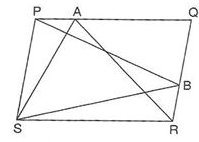Solution:

The area of triangle PBS = half the area of PQRS equation-1

the area of triangle ASR = half the area of PQRS equation-2

Reason is triangle and parallelogram on the same base and between the same parallel then triangle is half of parallelogram

the area of PBS + the area of ASR = half the area of PQRS + half the area of PQRS

the area of PBS + the area of ASR = the area of PQRS

the area of PBS + the area of ASR = 48cm2.

QUESTION: 2

Solution:
QUESTION: 3

### ABCD is a parallelogram. O is any point on diagonal BD. If ar(△DOP) = 8cm2, ar(△BOS) = 3cm2 and ar(△APS) = 6cm2,then ar(║ABCD) is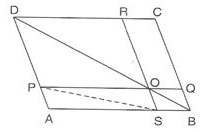Solution:
QUESTION: 4

ABC is a triangle in which D is the mid-point of BC. E and F are mid-points of DC and AE respectively. If ar(△ABC)=16cm2, then ar(△DEF) is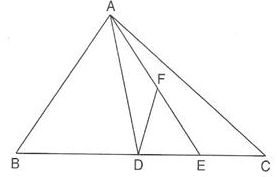Solution:
QUESTION: 5

The median of a triangle divides it into two

Solution:
QUESTION: 6

A, B, C, D are mid-points of sides of parallelogram PQRS. If ar(PQRS) = 36cm2, then ar (ABCD) is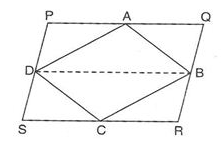Solution:
QUESTION: 7

PQRS is a trapezium with PQ║SR. A line parallel to PR intersects PQ at X and QR at Y. If ar(△PYR) = 5cm2,then ar(△PXS) is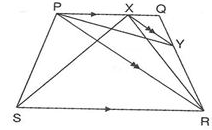Solution:
QUESTION: 8

In the given figure, ABCD is a rectangle and EFGH is a trapezium DE = CH. If ar(ABCD)=26cm2, then ar (EFGH) is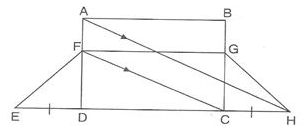Solution:
QUESTION: 9

If AD is median of △ABC and P is a point on AC such that ar(△ADP):ar(△ABD) = 2:3, then ar(△PDC) : ar(△ABC) is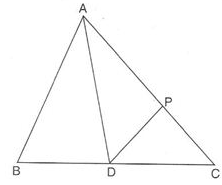Solution:
QUESTION: 10

ABCD is quadrilateral whose diagonal AC divides it into two parts, equal in area, then ABCD

Solution:
QUESTION: 11

ABCD is a trapezium in which AB║DC.If ar(△ABD) = 24cm2and AB = 8 cm, then the height of △ABC is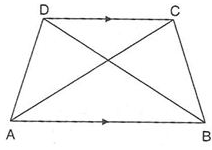Solution:
QUESTION: 12

D and E are mid-points of BC and AD respectively. If ar(△ABC) = 10cm2, then ar(△EBC)ar is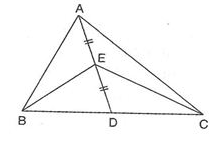Solution:
QUESTION: 13

ABCD, ABEF and AGHF are parallelograms. If ar(ABCD) = 23cm2, then ar (FGH) is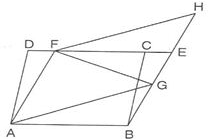Solution:
QUESTION: 14

The altitude of a parallelogram is twice the length of the base and its area is 1250cm2. The lengths of the base and the altitude respectively are :

Solution:
QUESTION: 15

Two parallelograms are on equal bases and between the same parallels. The ratio of their areas is

Solution:
QUESTION: 16

In the given figure if ar(║ABCD)=29cm2 and AB = 5.8 cm, then the height of ║ABEF is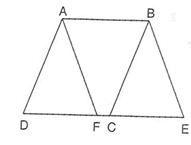Solution:
QUESTION: 17

Points A, B, C, and D are collinear. AB = BC = CD. XY║AD. If P and M lie on XY and ar(△MCD) = 7cm2,then ar(△APB) and ar(△APD) respectively are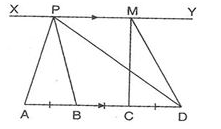Solution: Area of triangle APB=area of triangle CMD because these triangle have same base (AB=CD) and Between same parallel lines area of triangle =7cm^2.in triangle APD join PC then there are 3 triangle PAB, PBC andPCD whose areas are equal by a theorem. so area of triangle is three times area of triangle APB=3multiply7cm^2=21cm^2
QUESTION: 18

ABCD is a parallelogram in which DC is produced to P such that DC = CP. AP intersects BC at Q. If ar(△BQD) = 3cm2,then ar(║ABCD) is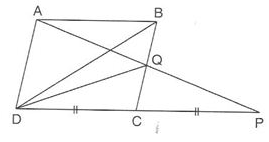Solution:
QUESTION: 19

ABCD is a parallelogram one of whose diagonals is AC. Then, which of the following is true?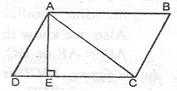Solution:
QUESTION: 20

If a triangle and a parallelogram are on the same base and between the same parallels, then the ratio of the area of the triangle to the area of the parallelogram is

Solution:
QUESTION: 21

AP ║ BQ ║ CR. If ar(△AQC)=17cm2, then ar(△PBR)ar(△PBR) is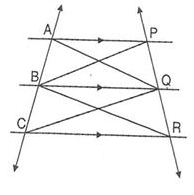Solution:
QUESTION: 22

If E and F are mid-points of sides AB and CD respectively and ar(║ABCD)=36cm2,thenar(APD):ar(DEF)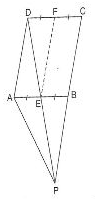Solution:
QUESTION: 23

PQRS is a parallelogram whose diagonals PR and SQ intersect at O. A line segment through O meets PQ at A and SR at B. If ar(PQRS) = 25cm2, then ar (SBAP) is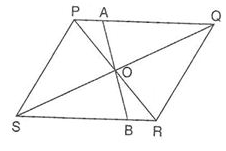Solution:
QUESTION: 24

In the figure, ABCD is a parallelogram and EFCD is rectangle. Now which of the following is correct option ?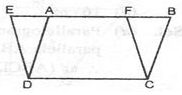Solution:
QUESTION: 25

Diagonals AC and BD of trapezium ABCD in which AB ║ DC, intersect each other at O. The triangle which is equal in area to triangle AOD is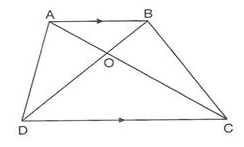Solution: